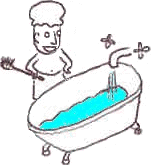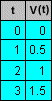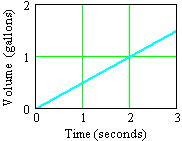Families of FunctionsWhen we wish to describe a particular function, we specify its input-output rule. We can do this in a number of ways. For example, if we wish to describe the volume of water in our bathtub, we might:

• Explain the behavior in plain language:

 "The volume starts (when t = 0 seconds) at V = 0 gallons, then increases at a constant rate of 0.5 gallons per second."

• Write down an equation:

V(t) = 0 + (0.5)t

• List a table of values:• Draw a graph:Each of these descriptions is a representation of the function that we are trying to model.